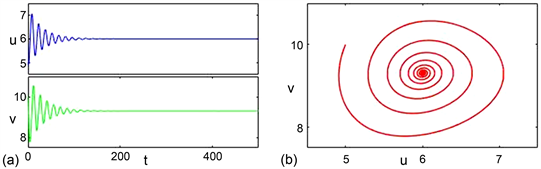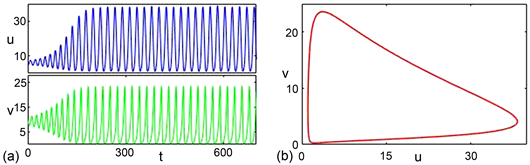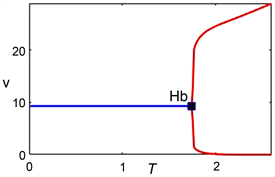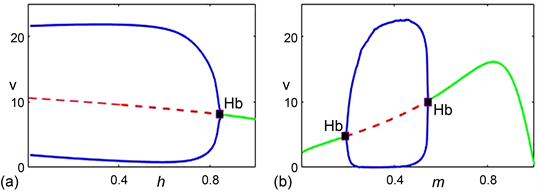# 一类带有时滞效应的修正的Leslie-Gower捕食者–食饵模型动力学分析Dynamics Analysis of a Modified Leslie-Gower Predator-Prey Model with Time Delay

DOI: 10.12677/AAM.2021.104131, PDF, HTML, XML, 下载: 12  浏览: 39

Abstract: This paper constructs a modified Leslie-Gower predator-prey model with Allee effect and prey refuge. The aim of this paper is to study the effect of time delay on the population dynamics. By using delay differential equation theory, we demonstrate the existence of Hopf bifurcation which is induced by time delay. According to center manifold theorem and normal form theory, the direction of Hopf bifurcation and stability of periodic solution are discussed. Additionally, numerical simulations reveal that the population density and the stability of positive equilibrium are sensitive for variations of time delay, Allee effect and prey refuge.

1. 引言

$\left\{\begin{array}{l}\frac{\text{d}u}{\text{d}t}=u\left({r}_{1}-{a}_{1}u-{c}_{1}v\right)\\ \frac{\text{d}v}{\text{d}t}=v\left({r}_{2}-\frac{{c}_{2}v}{u}\right)\end{array}$ (1.1)

$\left\{\begin{array}{l}\frac{\text{d}u}{\text{d}t}=u\left({r}_{1}-{a}_{1}u-\frac{h}{b+u}-{c}_{1}\left(1-m\right)v\right)\\ \frac{\text{d}v}{\text{d}t}=v\left({r}_{2}-\frac{{c}_{2}v\left(t-\tau \right)}{\left(1-m\right)u\left(t-\tau \right)+k}\right)\end{array}$ (1.2)

2. 动力学性质

2.1. 正平衡点的存在性与稳定性

$A=-{a}_{1}{c}_{2}-{c}_{1}{\left(1-m\right)}^{2}{r}_{2}$

$D=b{r}_{1}{c}_{2}-h{c}_{2}-b{c}_{1}k\left(1-m\right){r}_{2},$

$B={r}_{1}{c}_{2}-b{a}_{1}{c}_{2}-b{c}_{1}{\left(1-m\right)}^{2}{r}_{2}-{c}_{1}k\left(1-m\right){r}_{2}.$

${H}_{1}:m<\frac{h{c}_{2}+{c}_{1}k{r}_{2}-{r}_{1}{c}_{2}}{k{c}_{1}{r}_{2}},B>0,\Delta ={B}^{2}-4AD>0.$

${u}_{4}=\frac{-B+\sqrt{{B}^{2}-4AD}}{2A},{v}_{4}=\frac{{r}_{2}\left(\left(1-m\right){u}_{4}+k\right)}{{c}_{2}}$

${u}_{5}=\frac{-B-\sqrt{{B}^{2}-4AD}}{2A},{v}_{4}=\frac{{r}_{2}\left(\left(1-m\right){u}_{5}+k\right)}{{c}_{2}}$

${J}_{{E}_{i}}=\left(\begin{array}{cc}{u}_{i}\left(\frac{h}{{\left(b+{u}_{i}\right)}^{2}}-{a}_{1}\right)& -{c}_{1}\left(1-m\right){u}_{i}\\ \frac{{r}_{2}^{2}\left(1-m\right)}{{c}_{2}}& -{r}_{2}\end{array}\right),i=4,5$

$tr\left({J}_{{E}_{i}}\right)={u}_{i}\left(\frac{h}{{\left(b+{u}_{i}\right)}^{2}}-{a}_{1}\right)-{r}_{2},$ (2.1.1)

$det\left({J}_{{E}_{i}}\right)=\frac{{u}_{i}{r}_{2}}{{c}_{2}{\left(b+{u}_{i}\right)}^{2}}\left(\left({a}_{1}{c}_{2}+{c}_{1}{\left(1-m\right)}^{2}{r}_{2}\right){\left(b+{u}_{i}\right)}^{2}-h{c}_{2}\right).$ (2.1.2)

$G\left(u\right)=\left(\left({a}_{1}{c}_{2}+{c}_{1}{\left(1-m\right)}^{2}{r}_{2}\right){\left(b+{u}_{i}\right)}^{2}-h{c}_{2}\right),$

${P}_{1}=\frac{2bA+\sqrt{-4h{c}_{2}A}}{-2A}.$

$tr\left({J}_{{E}_{5}}\right)=\frac{-1}{8{\left(b+{u}_{5}\right)}^{2}{A}^{3}}\left[{L}_{1}-{L}_{2}\sqrt{{\left(E-bA\right)}^{2}+4h{c}_{2}A}\right],$

${L}_{1}=h\left[4{A}^{2}\left(\left(E+bA\right)+2{r}_{2}{c}_{2}+b{a}_{1}{c}_{2}\right)-12AE{a}_{1}{c}_{2}\right]+4{\left(E-bA\right)}^{2}\left(A{r}_{2}-{a}_{1}E\right),$

${L}_{2}=h\left[-4{A}^{2}+4A{a}_{1}{c}_{2}\right]+4\left(E-bA\right)\left({a}_{1}E-A{r}_{2}\right),$

$E={r}_{1}{c}_{2}-{c}_{1}k\left(1-m\right){r}_{2}.$

$A<0$ 可知 $tr\left({J}_{{E}_{5}}\right)$ 的符号与函数 ${L}_{1}-{L}_{2}\sqrt{{\left(E-bA\right)}^{2}+4h{c}_{2}A}$ 一致。

${h}_{1}=\frac{-4{\left(E-bA\right)}^{2}\left(A{r}_{2}-{a}_{1}E\right)}{4{A}^{2}\left(\left(E+bA\right)+2{r}_{2}{c}_{2}+b{a}_{1}{c}_{2}\right)-12AE{a}_{1}{a}_{2}},$

${h}_{2}=\frac{-4\left(E-bA\right)\left({a}_{1}E-A{r}_{2}\right)}{-4{A}^{2}+4A{a}_{1}{c}_{2}}.$

1) ${h}_{2}>{h}_{1}$

${L}_{1}^{2}-{L}_{2}^{2}\left({\left(E-bA\right)}^{2}+4h{c}_{2}A\right)={h}^{2}\left(-4A{c}_{2}{\left(4A{a}_{1}{c}_{2}-4{A}^{2}\right)}^{2}\right)+h{M}_{1}+{M}_{2},$

${M}_{1}=64{A}^{3}\left[\left({a}_{1}b-{r}_{2}\right)\left(E{a}_{1}-A{r}_{2}\right){c}_{2}^{2}+\left(b{A}^{2}\left({a}_{1}b+3{r}_{2}\right)-4EA\left({a}_{1}b+\frac{1}{4}{r}_{2}\right)+{E}^{2}{a}_{1}\right){c}_{2}+{A}^{2}Eb\right],$

${M}_{2}=64{A}^{6}{b}^{3}{r}_{2}-64{A}^{5}E{b}^{2}{r}_{2}-128{A}^{4}{E}^{2}b{r}_{2}+128{A}^{4}{E}^{2}b{r}_{2}-64{A}^{3}{E}^{3}{a}_{1}b.$

${\Delta }_{1}={M}_{1}^{2}+16{M}_{2}A{c}_{2}{\left(4A{a}_{1}{c}_{2}-4{A}^{2}\right)}^{2}.$

${h}_{3}=\frac{-{M}_{1}-\sqrt{{\Delta }_{1}}}{-8A{c}_{2}{\left(4A{a}_{1}{c}_{2}-4{A}^{2}\right)}^{2}},{h}_{4}=\frac{-{M}_{1}+\sqrt{{\Delta }_{1}}}{-8A{c}_{2}{\left(4A{a}_{1}{c}_{2}-4{A}^{2}\right)}^{2}}.$

2) ${h}_{2}<{h}_{1}$

i) ${M}_{1}>0$ ；ii) ${M}_{1}<0,{\Delta }_{1}<0$ ；iii) ${M}_{1}<0,{\Delta }_{1}>0,h\in \left(0,{h}_{3}\right)\cup \left({h}_{4},+\infty \right)$

a) ${h}_{2}>{h}_{1},0，(b) ${h}_{2}>{h}_{1},{h}_{1}0,{h}_{3}

c) ${h}_{2}<{h}_{1},0，(d) ${h}_{2}<{h}_{1},{M}_{1}>0,{h}_{2}

e) ${h}_{2}<{h}_{1},{M}_{1}<0,{h}_{2}0,h\in \left(0,{h}_{3}\right)\cup \left({h}_{4},+\infty \right)$

f) ${h}_{2}<{h}_{1},{M}_{1}<0,{h}_{2}

2.2. Hopf分岔

$\left\{\begin{array}{l}x\left(t\right)=u\left(t\right)-{u}_{5}\\ y\left(t\right)=v\left(t\right)-{v}_{5}\end{array}$

$\left\{\begin{array}{l}\frac{\text{d}x\left(t\right)}{\text{d}t}={a}_{11}x\left(t\right)+{a}_{12}y\left(t\right)\\ \frac{\text{d}y\left(t\right)}{\text{d}t}={a}_{21}x\left(t-\tau \right)+{a}_{22}y\left(t-\tau \right)+{a}_{23}y\left(t\right)\end{array}$ (2.2.1)

${a}_{11}={u}_{5}\left(\frac{h}{{\left(b+{u}_{5}\right)}^{2}}-{a}_{1}\right),{a}_{12}=-{c}_{1}\left(1-m\right){u}_{5},$

${a}_{21}=\frac{{r}_{2}^{2}\left(1-m\right)}{{c}_{2}},{a}_{22}=-{r}_{2},{a}_{23}=0.$

$\left[\begin{array}{cc}{a}_{11}& {a}_{12}\\ {a}_{21}{\text{e}}^{-\lambda \tau }& {a}_{22}{\text{e}}^{-\lambda \tau }\end{array}\right]$

${\lambda }^{2}-\left({a}_{11}+{a}_{22}{\text{e}}^{-\lambda \tau }\right)\lambda +\left({a}_{11}{a}_{22}-{a}_{12}{a}_{21}\right){\text{e}}^{-\lambda \tau }=0.$ (2.2.2)

$\left\{\begin{array}{l}-{\omega }^{2}\left(\tau \right)+{a}_{22}\omega \left(\tau \right)\mathrm{sin}\omega \tau +\left({a}_{11}{a}_{22}-{a}_{12}{a}_{21}\right)\mathrm{cos}\omega \tau =0\\ -{a}_{11}\omega \left(\tau \right)-{a}_{22}\omega \left(\tau \right)\mathrm{cos}\omega \tau +\left({a}_{11}{a}_{22}-{a}_{12}{a}_{21}\right)\mathrm{sin}\omega \tau =0\end{array}$ (2.2.3)

${\omega }^{4}\left(\tau \right)+\left({a}_{11}^{2}-{a}_{22}^{2}\right){\omega }^{2}\left(\tau \right)-{\left({a}_{11}{a}_{22}-{a}_{12}{a}_{21}\right)}^{2}=0.$ (2.2.4)

${\omega }_{0}=\sqrt{\frac{\left({a}_{22}^{2}-{a}_{11}^{2}\right)+\sqrt{{\left({a}_{22}^{2}-{a}_{11}^{2}\right)}^{2}+4{\left({a}_{11}{a}_{22}-{a}_{12}{a}_{21}\right)}^{2}}}{2}}$ .

$\mathrm{cos}{\omega }_{0}\tau =\frac{-{\omega }_{0}^{2}{a}_{21}{a}_{12}}{{a}_{22}^{2}{\omega }_{0}^{2}+{\left({a}_{11}{a}_{22}-{a}_{12}{a}_{21}\right)}^{2}},$

${\tau }_{j}=\frac{1}{{\omega }_{0}}\mathrm{arccos}\frac{-{\omega }_{0}^{2}{a}_{21}{a}_{12}}{{a}_{22}^{2}{\omega }_{0}^{2}+{\left({a}_{11}{a}_{22}-{a}_{12}{a}_{21}\right)}^{2}}+\frac{2j\pi }{{\omega }_{0}},j=0,1,2,\cdots .$

${\left(\frac{\text{d}\lambda }{\text{d}\tau }\right)}^{-1}=\left(\frac{2\lambda -{a}_{11}}{\lambda \left({a}_{11}\lambda -{\lambda }^{2}\right)}-\frac{\tau }{\lambda }-\frac{{a}_{22}}{\lambda \left({a}_{11}{a}_{22}-{a}_{12}{a}_{21}\right)-{a}_{22}{\lambda }^{2}}\right).$

$\tau ={\tau }_{0}$ 代入上述方程 ${\left(\frac{\text{d}\lambda }{\text{d}\tau }\right)}^{-1}$ 中，通过分离实部与虚部可得

${Re{\left(\frac{\text{d}\lambda }{\text{d}\tau }\right)}^{-1}|}_{\tau ={\tau }_{0}}=\frac{{a}_{11}^{2}{\left({a}_{11}{a}_{22}-{a}_{12}{a}_{21}\right)}^{2}+2{\omega }_{0}^{2}{\left({a}_{11}{a}_{22}-{a}_{12}{a}_{21}\right)}^{2}+{a}_{22}^{2}{\omega }_{0}^{4}}{\left({a}_{11}^{2}{\omega }_{0}^{2}+{\omega }_{0}^{4}\right)\left({\left({a}_{11}{a}_{22}-{a}_{12}{a}_{21}\right)}^{2}+{a}_{22}^{2}{\omega }_{0}^{2}\right)}>0.$

2.3. Hopf分岔方向

$x\left(t\right)=u\left(t\tau \right)-{u}_{5},y\left(t\right)=v\left(t\tau \right)-{v}_{5},$

$\tau ={\tau }_{0}+\delta ,\varphi ={\left(x,y\right)}^{\text{T}},{\varphi }_{t}\left(\theta \right)=\varphi \left(t+\theta \right),\theta \in \left[-1,0\right].$

$\left(\begin{array}{c}\frac{\text{d}x}{\text{d}t}\\ \frac{\text{d}y}{\text{d}t}\end{array}\right)=\tau \left({B}_{1}\left(\begin{array}{c}x\left(t\right)\\ y\left(t\right)\end{array}\right)+{B}_{2}\left(\begin{array}{c}x\left(t-1\right)\\ y\left(t-1\right)\end{array}\right)+f\right)$ (2.3.1)

${a}_{11}=-{a}_{1}{u}_{5}+\frac{h{u}_{5}}{{\left(b+{u}_{5}\right)}^{2}},{a}_{12}=-{c}_{1}\left(1-m\right){u}_{5},$

${a}_{21}=\frac{{r}_{2}^{2}\left(1-m\right)}{{c}_{2}},{a}_{22}=-{r}_{2}.$

${f}_{1}=\left(\frac{hb}{{\left(b+{u}_{5}\right)}^{3}}-{a}_{1}\right){x}^{2}\left(t\right)-{c}_{1}\left(1-m\right)x\left(t\right)y\left(t\right),$

$\begin{array}{c}{f}_{2}=-\frac{{\left(1-m\right)}^{2}{r}_{2}^{2}}{{c}_{2}\left(\left(1-m\right){u}_{5}+k\right)}{x}^{2}\left(t-1\right)+\frac{{r}_{2}\left(1-m\right)}{\left(1-m\right){u}_{5}+k}x\left(t-1\right)y\left(t\right)\\ \text{\hspace{0.17em}}+\frac{{r}_{2}\left(1-m\right)}{\left(1-m\right){u}_{5}+k}x\left(t-1\right)y\left(t-1\right)-\frac{{c}_{2}}{\left(1-m\right){u}_{5}+k}y\left(t\right)y\left(t-1\right).\end{array}$

${L}_{\delta }\left(\varphi \right)={\int }_{-1}^{0}\text{d}\eta \left(\theta ,\delta \right)\varphi \left(\theta \right),\varphi \in C\left(\left[-1,0\right],{R}^{2}\right).$

$\eta \left(\theta ,\delta \right)={\tau }_{0}+\delta \left[{B}_{1}\kappa \left(\theta \right)-{B}_{2}\kappa \left(\theta +1\right)\right].$

$\kappa \left(\theta \right)=\left\{\begin{array}{l}0\text{\hspace{0.17em}}\text{\hspace{0.17em}}\text{\hspace{0.17em}}\text{ }\text{ }\theta \ne 0\\ 1\text{\hspace{0.17em}}\text{\hspace{0.17em}}\text{\hspace{0.17em}}\text{\hspace{0.17em}}\theta =0\end{array}$

$P\left(\delta \right)\varphi \left(\theta \right)=\left\{\begin{array}{l}\frac{\text{d}\varphi \left(\theta \right)}{\text{d}\theta },\text{\hspace{0.17em}}\text{\hspace{0.17em}}\text{\hspace{0.17em}}\text{\hspace{0.17em}}\text{\hspace{0.17em}}\text{\hspace{0.17em}}\text{\hspace{0.17em}}\text{\hspace{0.17em}}\text{\hspace{0.17em}}\text{\hspace{0.17em}}\text{\hspace{0.17em}}\text{\hspace{0.17em}}\text{\hspace{0.17em}}\text{\hspace{0.17em}}\text{\hspace{0.17em}}-1\le \theta <0\\ {\int }_{-1}^{0}\text{d}\eta \left(s,\delta \right)\varphi \left(s\right),\text{\hspace{0.17em}}\text{\hspace{0.17em}}\text{\hspace{0.17em}}\text{\hspace{0.17em}}\text{\hspace{0.17em}}\theta =0\end{array}$

$Q\left(\delta \right)\varphi \left(\theta \right)=\left\{\begin{array}{l}0\text{\hspace{0.17em}}\text{\hspace{0.17em}}\text{\hspace{0.17em}}\text{\hspace{0.17em}}\text{\hspace{0.17em}}\text{\hspace{0.17em}}\text{\hspace{0.17em}}\text{\hspace{0.17em}}\text{\hspace{0.17em}}\text{\hspace{0.17em}}\text{\hspace{0.17em}}-1\le \theta <0\\ f\left(\delta \right)\varphi \text{\hspace{0.17em}}\text{\hspace{0.17em}}\text{\hspace{0.17em}}\text{\hspace{0.17em}}\text{\hspace{0.17em}}\text{\hspace{0.17em}}\theta =0\end{array}$

$\frac{\text{d}U}{\text{d}t}=P\left(\delta \right){U}_{t}+Q\left(\delta \right){U}_{t}$ (2.3.2)

$U={\left(x,y\right)}^{\text{T}},U\left(t\right)=U\left(t+\theta \right),\theta \in \left[-1,0\right]$

${P}^{*}\psi \left(s\right)=\left\{\begin{array}{l}-\frac{\text{d}\psi \left(s\right)}{\text{d}s},\text{\hspace{0.17em}}\text{\hspace{0.17em}}\text{\hspace{0.17em}}\text{\hspace{0.17em}}\text{\hspace{0.17em}}\text{\hspace{0.17em}}\text{\hspace{0.17em}}\text{\hspace{0.17em}}\text{\hspace{0.17em}}\text{\hspace{0.17em}}\text{\hspace{0.17em}}\text{\hspace{0.17em}}\text{\hspace{0.17em}}\text{\hspace{0.17em}}\text{\hspace{0.17em}}\text{\hspace{0.17em}}0

$〈\psi \left(s\right),\varphi \left(\theta \right)〉=\stackrel{¯}{\psi }\left(0\right)\varphi \left(0\right)-{\int }_{-1}^{0}{\int }_{\varsigma =0}^{\theta }\stackrel{¯}{\psi }\left(\varsigma -\theta \right)\text{d}\eta \left(\theta \right)\varphi \left(\varsigma \right).$

$P\left(0\right)$ 对应特征值 $i{\tau }_{0}{\omega }_{0}$ 的特征向量为 $q\left(\theta \right)$${P}^{*}$ 对应特征值 $-i{\tau }_{0}{\omega }_{0}$ 的特征向量为 ${q}^{*}\left( s \right)$

$q\left(\theta \right)={\left(1,\alpha \right)}^{\text{T}}{\text{e}}^{i{\omega }_{0}{\tau }_{0}\theta },{q}^{*}\left(s\right)=D{\left(1,\beta \right)}^{\text{T}}{\text{e}}^{i{\omega }_{0}{\tau }_{0}s}.$

$P\left(0\right)q\left(\theta \right)=q\left(\theta \right)i{\tau }_{0}{\omega }_{0}.$

$\left[i{\omega }_{0}I-\left({K}_{1}+{K}_{2}\right){\text{e}}^{-i{\omega }_{0}{\tau }_{0}}\right]q\left(0\right)=0.$

$\left[\begin{array}{cc}i{\omega }_{0}-{a}_{11}& -{a}_{12}\\ -{a}_{21}{\text{e}}^{-i{\omega }_{0}{\tau }_{0}}& i{\omega }_{0}-{a}_{22}{\text{e}}^{-i{\omega }_{0}{\tau }_{0}}\end{array}\right]\left(\begin{array}{c}1\\ \alpha \end{array}\right)=\left(\begin{array}{c}0\\ 0\end{array}\right),$

$\left[\begin{array}{cc}i{\omega }_{0}+{a}_{11}& {a}_{21}{\text{e}}^{-i{\omega }_{0}{\tau }_{0}}\\ {a}_{12}& i{\omega }_{0}+{a}_{22}{\text{e}}^{-i{\omega }_{0}{\tau }_{0}}\end{array}\right]\left(\begin{array}{c}1\\ \beta \end{array}\right)=\left(\begin{array}{c}0\\ 0\end{array}\right).$

$\alpha =\frac{i{\omega }_{0}-{a}_{11}}{{a}_{12}},\beta =-\frac{\left(i{\omega }_{0}+{a}_{11}\right){\text{e}}^{i{\tau }_{0}{\omega }_{0}}}{{a}_{21}}.$

$\begin{array}{c}{q}^{*}\left(s\right),q\left(\theta \right)={\stackrel{¯}{q}}^{*}\left(0\right)q\left(0\right)-{\int }_{-1}^{0}{\int }_{0}^{\theta }{\stackrel{¯}{q}}^{*}\left(\varsigma -\theta \right)\text{d}\eta \left(\theta \right)q\left(\varsigma \right)\text{d}\varsigma \\ =\stackrel{¯}{D}\left(\begin{array}{cc}1& \stackrel{¯}{\beta }\end{array}\right)\left(\begin{array}{c}1\\ \alpha \end{array}\right)-{\int }_{-1}^{0}{\int }_{0}^{\theta }\stackrel{¯}{D}\left(\begin{array}{cc}1& \stackrel{¯}{\beta }\end{array}\right){\text{e}}^{-i{\omega }_{0}{\tau }_{0}\left(\varsigma -\theta \right)}\text{d}\eta \left(\theta \right)\left(\begin{array}{c}1\\ \alpha \end{array}\right){\text{e}}^{-i{\omega }_{0}{\tau }_{0}\varsigma }\text{d}\varsigma \\ =\stackrel{¯}{D}\left(1+\alpha \stackrel{¯}{\beta }\right)+{\tau }_{0}\stackrel{¯}{D}{\text{e}}^{-i{\tau }_{0}{\omega }_{0}}\left(\frac{\stackrel{¯}{\beta }{r}_{2}^{2}\left(1-m\right)}{{c}_{2}}-\stackrel{¯}{\beta }{r}_{2}\alpha \right).\end{array}$

$D={\left(1+\stackrel{¯}{\alpha }\beta +{\tau }_{\text{0}}{\text{e}}^{i{\tau }_{0}{\omega }_{0}}\left(\frac{\beta {r}_{2}^{2}\left(1-m\right)}{{c}_{2}}-\beta {r}_{2}\stackrel{¯}{\alpha }\right)\right)}^{-1},$

$〈{q}^{*}\left(s\right),q\left(\theta \right)〉=1,〈{q}^{*}\left(s\right),\stackrel{¯}{q}\left(\theta \right)〉=0.$

$z\left(t\right)=〈{q}^{*},{U}_{t}〉,W\left(t,\theta \right)={U}_{t}\left(\theta \right)-2Re\left\{z\left(t\right)q\left(\theta \right)\right\},$ (2.3.3)

$W\left(t,\theta \right)=W\left(z,\stackrel{¯}{z},\theta \right)={W}_{20}\left(\theta \right)\frac{{z}^{2}}{2}+{W}_{11}\left(\theta \right)z\stackrel{¯}{z}+{W}_{02}\left(\theta \right)\frac{{\stackrel{¯}{z}}^{2}}{2}+{W}_{30}\left(\theta \right)\frac{{z}^{3}}{6}+\cdots$ (2.3.4)

$\delta =0$ 时，对于 ${U}_{t}\in {C}_{0}$，有下式成立：

$\begin{array}{l}\frac{\text{d}z\left(t\right)}{\text{d}t}=〈{q}^{*},\stackrel{˙}{U}\left(t\right)〉=i{\omega }_{0}{\tau }_{0}z\left(t\right)+〈{\stackrel{¯}{q}}^{*}\left(\theta \right),Q\left(0\right)\left(W\left(z,\stackrel{¯}{z},\theta \right)+2Re\left\{z\left(t\right)q\left(\theta \right)\right\}\right)〉\\ ={\stackrel{¯}{q}}^{*}\left(0\right)f\left(0\right)\left(W\left(z,\stackrel{¯}{z},0\right)+2Re\left\{z\left(t\right)q\left(0\right)\right\}\right)\\ \triangleq i{\omega }_{0}{\tau }_{0}z\left(t\right)+g\left(z,\stackrel{¯}{z}\right).\end{array}$

$\begin{array}{c}g\left(z,\stackrel{¯}{z}\right)={\stackrel{¯}{q}}^{*}\left(0\right)f\left(0\right)\left(W\left(z,\stackrel{¯}{z},0\right)+2Re\left\{z\left(t\right)q\left(0\right)\right\}\right)=\stackrel{¯}{D}{\tau }_{0}\left({f}_{1}+\stackrel{¯}{\beta }{f}_{2}\right)\\ ={g}_{20}\frac{{z}^{2}}{2}+{g}_{11}z\stackrel{¯}{z}+{g}_{02}\frac{{\stackrel{¯}{z}}^{2}}{2}+{g}_{21}\frac{{z}^{2}\stackrel{¯}{z}}{2}+\cdots \end{array}$ (2.3.5)

$q\left(0\right)={\left(1,\alpha \right)}^{\text{T}},$

$\begin{array}{c}{U}_{t}\left(\theta \right)={\left({x}_{t}\left(\theta \right),{y}_{t}\left(\theta \right)\right)}^{\text{T}}=2Re\left\{z\left(t\right)q\left(\theta \right)\right\}+W\left(t,\theta \right)\\ =zq\left(\theta \right)+\stackrel{¯}{z}\text{ }\stackrel{¯}{q}\left(\theta \right)+W\left(t,\theta \right).\end{array}$

$x\left(t\right)=z+\stackrel{¯}{z}+{W}_{20}^{\left(1\right)}\left(0\right)\frac{{z}^{2}}{2}+{W}_{11}^{\left(1\right)}\left(0\right)z\stackrel{¯}{z}+{W}_{02}^{\left(1\right)}\left(0\right)\frac{{\stackrel{¯}{z}}^{2}}{2},$

$y\left(t\right)=\alpha z+\stackrel{¯}{\alpha }\stackrel{¯}{z}+{W}_{20}^{\left(2\right)}\left(0\right)\frac{{z}^{2}}{2}+{W}_{11}^{\left(2\right)}\left(0\right)z\stackrel{¯}{z}+{W}_{02}^{\left(2\right)}\left(0\right)\frac{{\stackrel{¯}{z}}^{2}}{2},$

$x\left(t-1\right)=z{\text{e}}^{-i{\omega }_{0}{\tau }_{0}}+\stackrel{¯}{z}{\text{e}}^{i{\omega }_{0}{\tau }_{0}}+{W}_{20}^{\left(1\right)}\left(-1\right)\frac{{z}^{2}}{2}+{W}_{11}^{\left(1\right)}\left(-1\right)z\stackrel{¯}{z}+{W}_{02}^{\left(1\right)}\left(-1\right)\frac{{\stackrel{¯}{z}}^{2}}{2},$

$y\left(t-1\right)=\alpha z{\text{e}}^{-i{\omega }_{0}{\tau }_{0}}+\stackrel{¯}{\alpha }\stackrel{¯}{z}{\text{e}}^{i{\omega }_{0}{\tau }_{0}}+{W}_{20}^{\left(2\right)}\left(-1\right)\frac{{z}^{2}}{2}+{W}_{11}^{\left(2\right)}\left(-1\right)z\stackrel{¯}{z}+{W}_{02}^{\left(2\right)}\left(-1\right)\frac{{\stackrel{¯}{z}}^{2}}{2}.$

${g}_{20}=2\stackrel{¯}{D}{\tau }_{0}\left[{f}_{11}+{f}_{12}\alpha +{f}_{21}\stackrel{¯}{\beta }{\text{e}}^{-2i{\omega }_{0}{\tau }_{0}}+{f}_{22}\stackrel{¯}{\beta }\alpha {\text{e}}^{-i{\omega }_{0}{\tau }_{0}}+{f}_{23}\stackrel{¯}{\beta }\alpha {\text{e}}^{-2i{\omega }_{0}{\tau }_{0}}+{f}_{24}{\alpha }^{2}\stackrel{¯}{\beta }{\text{e}}^{-i{\omega }_{0}{\tau }_{0}}\right],$

$\begin{array}{c}{g}_{11}=\stackrel{¯}{D}{\tau }_{0}\left[2{f}_{11}+{f}_{12}\left(\stackrel{¯}{\alpha }+\alpha \right)+2{f}_{21}\stackrel{¯}{\beta }+{f}_{22}\stackrel{¯}{\beta }\left(\stackrel{¯}{\alpha }{\text{e}}^{-i{\omega }_{0}{\tau }_{0}}+\alpha {\text{e}}^{i{\omega }_{0}{\tau }_{0}}\right)\\ \text{\hspace{0.17em}}+{f}_{23}\stackrel{¯}{\beta }\left(\stackrel{¯}{\alpha }+\alpha \right)+{f}_{24}\stackrel{¯}{\beta }\left(\alpha \stackrel{¯}{\alpha }{\text{e}}^{i{\omega }_{0}{\tau }_{0}}+\alpha \stackrel{¯}{\alpha }{\text{e}}^{-i{\omega }_{0}{\tau }_{0}}\right)\right],\end{array}$

${g}_{02}=2\stackrel{¯}{D}{\tau }_{0}\left[{f}_{11}+{f}_{12}\stackrel{¯}{\alpha }+{f}_{21}\stackrel{¯}{\beta }{\text{e}}^{2i{\omega }_{0}{\tau }_{0}}+{f}_{22}\stackrel{¯}{\beta }\stackrel{¯}{\alpha }{\text{e}}^{i{\omega }_{0}{\tau }_{0}}+{f}_{23}\stackrel{¯}{\beta }\stackrel{¯}{\alpha }{\text{e}}^{2i{\omega }_{0}{\tau }_{0}}+{f}_{24}{\stackrel{¯}{\alpha }}^{2}\stackrel{¯}{\beta }{\text{e}}^{i{\omega }_{0}{\tau }_{0}}\right],$

$\begin{array}{c}{g}_{21}=2\stackrel{¯}{D}{\tau }_{0}\left[{f}_{11}\left({W}_{20}^{1}\left(0\right)+2{W}_{11}^{1}\left(0\right)\right)+{f}_{12}\left({W}_{11}^{2}\left(0\right)+\frac{1}{2}{W}_{20}^{2}\left(0\right)+\frac{1}{2}{W}_{20}^{1}\left(0\right)\stackrel{¯}{\alpha }+{W}_{11}^{1}\left(0\right)\alpha \right)\\ \text{\hspace{0.17em}}+\stackrel{¯}{\beta }\left({f}_{21}\left({W}_{20}^{1}\left(-1\right){\text{e}}^{i{\omega }_{0}{\tau }_{0}}+2{W}_{11}^{1}\left(0\right){\text{e}}^{-i{\omega }_{0}{\tau }_{0}}\right)\right)\\ \text{\hspace{0.17em}}+{f}_{22}\left(\frac{1}{2}{W}_{20}^{2}\left(0\right){\text{e}}^{i{\omega }_{0}{\tau }_{0}}+{W}_{11}^{2}\left(0\right){\text{e}}^{-i{\omega }_{0}{\tau }_{0}}+\frac{1}{2}{W}_{20}^{1}\left(-1\right)\stackrel{¯}{\alpha }+\alpha {W}_{11}^{1}\left(-1\right)\right)\\ \text{\hspace{0.17em}}+{f}_{23}\left(\frac{1}{2}{W}_{20}^{2}\left(-1\right){\text{e}}^{i{\omega }_{0}{\tau }_{0}}+{W}_{11}^{2}\left(-1\right){\text{e}}^{-i{\omega }_{0}{\tau }_{0}}+\frac{1}{2}{W}_{20}^{1}\left(-1\right)\stackrel{¯}{\alpha }{\text{e}}^{i{\omega }_{0}{\tau }_{0}}+{W}_{11}^{1}\left(-1\right)\alpha {\text{e}}^{-i{\omega }_{0}{\tau }_{0}}\right)\\ \text{\hspace{0.17em}}+{f}_{24}\left(\frac{1}{2}{W}_{20}^{2}\left(0\right)\stackrel{¯}{\alpha }{\text{e}}^{i{\omega }_{0}{\tau }_{0}}+{W}_{11}^{2}\left(0\right)\alpha {\text{e}}^{-i{\omega }_{0}{\tau }_{0}}+\alpha {W}_{11}^{2}\left(-1\right)+\frac{1}{2}\stackrel{¯}{\alpha }{W}_{20}^{2}\left(-1\right)\right)\right],\end{array}$

${f}_{11}=\left(\frac{hb}{{\left(b+{u}_{5}\right)}^{3}}-{a}_{1}\right),{f}_{12}=-{c}_{1}\left(1-m\right),{f}_{21}=\frac{{\left(1-m\right)}^{2}{r}_{2}^{2}}{{c}_{2}\left({u}_{5}\left(1-m\right)+k\right)},$

${f}_{22}=\frac{{r}_{2}\left(1-m\right)}{{u}_{5}\left(1-m\right)+k},{f}_{23}=\frac{{r}_{2}\left(1-m\right)}{{u}_{5}\left(1-m\right)+k},{f}_{24}=-\frac{{c}_{2}}{{u}_{5}\left(1-m\right)+k}.$

$\begin{array}{c}\stackrel{˙}{W}={\stackrel{˙}{U}}_{t}-2Re\left\{\stackrel{˙}{z}q\right\}={\stackrel{˙}{U}}_{t}-\stackrel{˙}{z}q-\stackrel{˙}{\stackrel{¯}{z}}\text{ }\stackrel{¯}{q}=P{U}_{t}+Q{U}_{t}-\stackrel{˙}{z}q-\stackrel{˙}{\stackrel{¯}{z}}\text{ }\stackrel{¯}{q}\\ =\left\{\begin{array}{l}PW-2Re\left\{{\stackrel{¯}{q}}^{*}\left(\theta \right)f\left(0\right)q\left(\theta \right)\right\},\text{\hspace{0.17em}}\text{\hspace{0.17em}}\text{\hspace{0.17em}}\text{\hspace{0.17em}}\text{\hspace{0.17em}}\text{\hspace{0.17em}}\text{\hspace{0.17em}}\text{\hspace{0.17em}}\text{\hspace{0.17em}}\text{\hspace{0.17em}}\text{\hspace{0.17em}}\text{\hspace{0.17em}}0<\theta \le 1\\ PW-2Re\left\{{\stackrel{¯}{q}}^{*}\left(0\right)f\left(0\right)q\left(0\right)\right\}+f\left(0\right),\text{\hspace{0.17em}}\text{\hspace{0.17em}}\text{\hspace{0.17em}}\text{\hspace{0.17em}}\text{\hspace{0.17em}}\text{\hspace{0.17em}}\theta =0\end{array}\\ =PW+H\left(z,\stackrel{¯}{z},\theta \right)\end{array}$

$H\left(z,\stackrel{¯}{z},\theta \right)=\frac{{H}_{20}\left(\theta \right){z}^{2}}{2}+{H}_{11}\left(\theta \right)z\stackrel{¯}{z}+\frac{{H}_{02}\left(\theta \right){\stackrel{¯}{z}}^{2}}{2}+\cdots .$

$\begin{array}{c}\stackrel{˙}{W}\left(z,\stackrel{¯}{z}\right)={W}_{z}\stackrel{˙}{z}+{W}_{\stackrel{\stackrel{¯}{z}}{z}}\stackrel{˙}{\stackrel{¯}{z}}={W}_{20}\left(\theta \right)z\stackrel{˙}{z}+{W}_{11}\left(\theta \right)\stackrel{¯}{z}\stackrel{˙}{z}+{W}_{02}\stackrel{¯}{z}\text{ }\stackrel{˙}{\stackrel{¯}{z}}+{W}_{11}\left(\theta \right)z\stackrel{˙}{\stackrel{¯}{z}}+O\left({|\left(z,\stackrel{¯}{z}\right)|}^{3}\right)\\ ={W}_{20}\left(\theta \right)z\left(i{\omega }_{0}{\tau }_{0}z+g\left(z,\stackrel{¯}{z}\right)\right)+{W}_{11}\left(\theta \right)\stackrel{¯}{z}\left(i{\omega }_{0}{\tau }_{0}\stackrel{¯}{z}+\stackrel{¯}{g}\left(z,\stackrel{¯}{z}\right)\right)\\ \text{\hspace{0.17em}}+{W}_{11}z\left(-i{\omega }_{0}{\tau }_{0}\stackrel{¯}{z}+\stackrel{¯}{g}\left(z,\stackrel{¯}{z}\right)\right)+{W}_{02}\left(\theta \right)\stackrel{¯}{z}\left(-i{\omega }_{0}{\tau }_{0}\stackrel{¯}{z}+\stackrel{¯}{g}\left(z,\stackrel{¯}{z}\right)\right)+O\left({|\left(z,\stackrel{¯}{z}\right)|}^{3}\right)\\ =i{\omega }_{0}{\tau }_{0}{W}_{20}\left(\theta \right){z}^{2}-i{\omega }_{0}{\tau }_{0}{W}_{02}\left(\theta \right){\stackrel{¯}{z}}^{2}+O\left({|\left(z,\stackrel{¯}{z}\right)|}^{3}\right)\end{array}$

$\left(2i{\omega }_{0}{\tau }_{0}I-P\left(0\right)\right){W}_{20}\left(\theta \right)={H}_{20}\left(\theta \right),$

$P\left(0\right){W}_{11}\left(\theta \right)=-{H}_{11}\left(\theta \right),$ (2.3.6)

$\left(2i{\omega }_{0}{\tau }_{0}I+P\left(0\right)\right){W}_{02}\left(\theta \right)=-{H}_{02}\left( \theta \right)$

$\begin{array}{c}H\left(z,\stackrel{¯}{z},\theta \right)=-2Re\left\{{\stackrel{¯}{q}}^{*}\left(\theta \right)Q\left(0\right)q\left(\theta \right)\right\}=-g\left(z,\stackrel{¯}{z}\right)q\left(\theta \right)-\stackrel{¯}{g}\left(z,\stackrel{¯}{z}\right)\stackrel{¯}{q}\left(\theta \right)\\ =-\left({g}_{20}\frac{{z}^{2}}{2}+{g}_{11}z\stackrel{¯}{z}+{g}_{02}\frac{{\stackrel{¯}{z}}^{2}}{2}+\cdots \right)q\left(\theta \right)-\left({\stackrel{¯}{g}}_{20}\frac{{\stackrel{¯}{z}}^{2}}{2}+{\stackrel{¯}{g}}_{11}z\stackrel{¯}{z}+{\stackrel{¯}{g}}_{02}\frac{{\stackrel{¯}{z}}^{2}}{2}+\cdots \right)\stackrel{¯}{q}\left(\theta \right).\end{array}$

${H}_{20}\left(\theta \right)=-{g}_{20}q\left(\theta \right)-{\stackrel{¯}{g}}_{02}\stackrel{¯}{q}\left(\theta \right),$

${H}_{11}\left(\theta \right)=-{g}_{11}q\left(\theta \right)-{\stackrel{¯}{g}}_{11}\stackrel{¯}{q}\left(\theta \right).$ (2.3.7)

$\stackrel{̇}{{W}_{20}}\left(\theta \right)=2i{\omega }_{0}{\tau }_{0}{W}_{20}\left(\theta \right)+{g}_{20}q\left(\theta \right)+{\stackrel{¯}{g}}_{02}\stackrel{¯}{q}\left(\theta \right),$

${W}_{20}\left(\theta \right)=\frac{i{g}_{20}q\left(0\right)}{{\omega }_{0}{\tau }_{0}}{\text{e}}^{i{\omega }_{0}{\tau }_{0}\theta }+\frac{i{\stackrel{¯}{g}}_{02}\stackrel{¯}{q}\left(0\right)}{3{\omega }_{0}{\tau }_{0}}{\text{e}}^{-i{\omega }_{0}{\tau }_{0}\theta }+{G}_{1}{\text{e}}^{2i{\omega }_{0}{\tau }_{0}\theta },$

${W}_{11}\left(\theta \right)=\frac{-i{g}_{11}q\left(0\right)}{{\omega }_{0}{\tau }_{0}}{\text{e}}^{i{\omega }_{0}{\tau }_{0}\theta }+\frac{i{\stackrel{¯}{g}}_{11}\stackrel{¯}{q}\left(0\right)}{{\omega }_{0}{\tau }_{0}}{\text{e}}^{-i{\omega }_{0}{\tau }_{0}\theta }+{G}_{2}.$

$\theta =0$ 时，有

${H}_{20}\left(0\right)=-{g}_{20}q\left(0\right)-{\stackrel{¯}{g}}_{02}\stackrel{¯}{q}\left(0\right)+{\tau }_{0}{\left({z}_{1},{z}_{2},0\right)}^{\text{T}},$

${H}_{11}\left(0\right)=-{g}_{11}q\left(0\right)-{\stackrel{¯}{g}}_{11}\stackrel{¯}{q}\left(0\right)+{\tau }_{0}{\left({z}_{3},{z}_{4},0\right)}^{\text{T}}.$

${z}_{1}={f}_{11}+{f}_{12}\alpha ,$

${z}_{2}={f}_{21}{\text{e}}^{-2i{\omega }_{0}{\tau }_{0}}+{f}_{22}{\text{e}}^{-i{\omega }_{0}{\tau }_{0}}\alpha +{f}_{23}\alpha {\text{e}}^{-2i{\omega }_{0}{\tau }_{0}}+{f}_{24}{\alpha }^{2}{\text{e}}^{-i{\omega }_{0}{\tau }_{0}},$

${z}_{3}=2{f}_{11}+{f}_{12}\left(\alpha +\stackrel{¯}{\alpha }\right),$

${z}_{4}=2{f}_{21}+{f}_{22}\left({\text{e}}^{-i{\omega }_{0}{\tau }_{0}}\stackrel{¯}{\alpha }+{\text{e}}^{i{\omega }_{0}{\tau }_{0}}\alpha \right)+{f}_{23}\left(\alpha +\stackrel{¯}{\alpha }\right)+{f}_{24}\left(\alpha \stackrel{¯}{\alpha }{\text{e}}^{-i{\omega }_{0}{\tau }_{0}}+\alpha \stackrel{¯}{\alpha }{\text{e}}^{i{\omega }_{0}{\tau }_{0}}\right).$

${\int }_{-1}^{0}\text{d}\eta \left(\theta \right){W}_{20}\left(\theta \right)=2i{\omega }_{0}{\tau }_{0}{W}_{20}\left(0\right)-{H}_{20}\left(0\right),{\int }_{-1}^{0}\text{d}\eta \left(\theta \right){W}_{11}\left(\theta \right)=-{H}_{11}\left(0\right).$

${H}_{20}\left(0\right)=2i{\omega }_{0}{\tau }_{0}{W}_{20}\left(0\right)-{\tau }_{0}{B}_{1}{W}_{20}\left(0\right)-{\tau }_{0}{B}_{2}{W}_{20}\left(-1\right),$

${H}_{11}\left(0\right)=-{\tau }_{0}{B}_{1}{W}_{11}\left(0\right)-{\tau }_{0}{B}_{2}{W}_{11}\left(-1\right).$

$\begin{array}{c}{G}_{1}=\frac{1}{2i{\omega }_{0}{\tau }_{0}I-{\tau }_{0}{B}_{1}-{\tau }_{0}{B}_{2}{\text{e}}^{-2i{\omega }_{0}{\tau }_{0}}}\left({H}_{20}\left(0\right)+2{g}_{20}q\left(0\right)+\frac{2}{3}{\stackrel{¯}{g}}_{02}\stackrel{¯}{q}\left(0\right)+\frac{{B}_{1}i{g}_{20}q\left(0\right)}{{\omega }_{0}}\\ \text{\hspace{0.17em}}+\frac{{B}_{1}i{\stackrel{¯}{g}}_{02}\stackrel{¯}{q}\left(0\right)}{3{\omega }_{0}}+{B}_{2}\frac{i{g}_{20}q\left(0\right)}{{\omega }_{0}}{\text{e}}^{-i{\omega }_{0}{\tau }_{0}}+{B}_{2}\frac{i{\stackrel{¯}{g}}_{02}\stackrel{¯}{q}\left(0\right)}{3{\omega }_{0}}{\text{e}}^{i{\omega }_{0}{\tau }_{0}}\right),\end{array}$

${G}_{2}=\frac{1}{{\tau }_{0}\left({B}_{1}+{B}_{2}\right)}\left(-{H}_{11}\left(0\right)+\frac{{B}_{1}i{g}_{11}q\left(0\right)}{{\omega }_{0}}-\frac{{B}_{1}i{\stackrel{¯}{g}}_{11}\stackrel{¯}{q}\left(0\right)}{{\omega }_{0}}+\frac{{B}_{2}i{g}_{11}q\left(0\right)}{{\omega }_{0}}{\text{e}}^{-i{\omega }_{0}{\tau }_{0}}-\frac{{B}_{2}i{\stackrel{¯}{g}}_{11}\stackrel{¯}{q}\left(0\right)}{{\omega }_{0}}{\text{e}}^{i{\omega }_{0}{\tau }_{0}}\right).$

${c}_{1}\left(0\right)=\frac{i}{2{\omega }_{0}{\tau }_{0}}\left({g}_{20}{g}_{11}-2{|{g}_{11}|}^{2}-\frac{{|{g}_{02}|}^{2}}{3}\right)+\frac{{g}_{21}}{2},$

${\beta }_{2}=Re\left({c}_{1}\left(0\right)\right),{\mu }_{2}=-\frac{Re\left({c}_{1}\left(0\right)\right)}{Re\left({\lambda }^{\prime }\left({\tau }_{0}\right)\right)},$

${T}_{2}=-\frac{Im\left\{{c}_{1}\left(0\right)\right\}+{\mu }_{2}Im\left\{{\lambda }^{\prime }\left({\tau }_{0}\right)\right\}}{{\omega }_{0}{\tau }_{0}}.$

3. 数值模拟

${r}_{1}=0.6,{r}_{2}=0.3,{c}_{1}=0.1,{c}_{2}=0.1,k=0.1,{a}_{1}=0.01,b=0.7.$Figure 1. $m=0.5,h=0.5,\tau =1.5$. (a) Time series; (b) Phase portraitFigure 2. $m=0.5,h=0.5,\tau =1.9$. (a) Time series; (b) Phase portraitFigure 3. Analysis of sensitivity with $m=0.5,h=0.5$. The solid blue line denotes the stable equilibrium; the solid red line represents the maximal and minimal density of predator population. The point “Hb” is the Hopf bifurcation pointFigure 4. (a) Analysis of sensitivity with $m=0.5,\tau =1.8$ ; (b) Analysis of sensitivity with $h=0.5,\tau =1.8$. The solid green line denotes the stable equilibrium; the dashed red line indicates the positive equilibrium which is unstable. The solid blue lines represent the maximal and minimal density of predator populations. The point “Hb” is the Hopf bifurcation point

4. 结论

  Kuang, Y. and Beretta, E. (1998) Global Qualitative of a Ratio-Dependent Predator-Prey System. Journal of Mathematical Biology, 36, 389-406. https://doi.org/10.1007/s002850050105  Freedman, H.I. and Mathsen, R.M. (1993) Persistence in Predator-Prey Systems with Ratio-Dependent Predator Influence. Bulletin of Mathematical Biology, 55, 817-827. https://doi.org/10.1016/S0092-8240(05)80190-9  Wang, J.F., Wei, J.J. and Shi, J.P. (2016) Global Bifurcation Analysis and Pattern Formation in Homogeneous Diffusive Predator-Prey. Journal of Differential Equations, 260, 3495-3523. https://doi.org/10.1016/j.jde.2015.10.036  潘陈蓉, 陈松林. 具有广义Holling III型功能反应的Leslie捕食-食饵系统的平衡点分类和稳定性分析[J]. 应用数学进展, 2019, 8(10): 1625-1631.  Leslie, P.H. (1948) Some Further Notes on the Use of Matrices in Population Mathematics. Biometrika, 35, 213-245. https://doi.org/10.1093/biomet/35.3-4.213  Leslie, P.H. and Gower, J.C. (1960) The Properties of a Stochastic Model for the Predator-Prey Type of Interaction between Two Species. Biometrica, 47, 219-234. https://doi.org/10.1093/biomet/47.3-4.219  Li, Y. and Xiao, D.M. (2007) Bifurcation of a Predator-Prey System of Holling and Leslie Types. Chaos Solitons Fractals, 34, 606-620. https://doi.org/10.1016/j.chaos.2006.03.068  Liu, M. and Wang, K. (2013) Dynamics of a Leslie-Gower Holling-Type II Predator-Prey System with Lévy Jumps. Nonlinear Analysis: Theory, Methods and Applications, 85, 204-213. https://doi.org/10.1016/j.na.2013.02.018  Huang, J.C., Ruan, S.G. and Song, J. (2014) Bifurcations in a Predator-Prey System of Leslie Type with Generalized Hollingtype III Functional Response. Journal of Differential Equations, 257, 1721-1752. https://doi.org/10.1016/j.jde.2014.04.024  Aziz-Alaoui, M.A. and Okiye, M.D. (2003) Boundedness and Global Stability for a Predator Prey Model with Modified Leslie Gower and Holling Type II Schemes. Applied Mathematics Letters, 16, 1069-1075. https://doi.org/10.1016/S0893-9659(03)90096-6  Gupta, R.P. and Chandra, P. (2013) Bifurcation Analysis of Modified Leslie-Gower Predator-Prey Model with Michaelis-Menten Type Prey Harvesting. Journal of Mathematical Analysis and Applications, 398, 278-295. https://doi.org/10.1016/j.jmaa.2012.08.057  Pal, P.J. and Mandal, P.K. (2014) Bifurcation Analysis of a Modified Leslie-Gower Predator-Prey Model with Beddington-DeAngelis Functional Response and Strong Allee Effect. Mathematical and Computers in Simulation, 97, 123-146. https://doi.org/10.1016/j.matcom.2013.08.007  Peng, F. and Yun, K. (2015) Dynamics of a Modified Leslie-Gower Model with Double Allee Effects. Nonlinear Dynamics, 80, 1051-1062. https://doi.org/10.1007/s11071-015-1927-2  Song, X.Y. and Li, Y.F. (2008) Dynamic Behaviors of the Periodic Predator-Prey Model with Modified Leslie-GowerHolling-Type II Schemes and Impulsive Effect. Nonlinear Analysis: Real World Applications, 9, 64-79. https://doi.org/10.1016/j.nonrwa.2006.09.004  Ji, C.Y., Jiang, D.Q. and Shi, N.Z. (2009) Analysis of a Predator-Prey Model with Modified Leslie-Gower and Holling-Type IIschemes with Stochastic Perturbation. SIAM Journal on Applied Mathematics, 359, 482-498. https://doi.org/10.1016/j.jmaa.2009.05.039  Nie, L.N., Teng, Z.D., Hu, L., et al. (2010) Qualitative Analysis of a Modified Leslie-Gower and Holling-Type II Predator-Prey Model with State Dependent Impulsive Effects. Nonlinear Analysis: Real World Applications, 11, 1364-1373. https://doi.org/10.1016/j.nonrwa.2009.02.026  Tian, Y.L. and Weng, P.X. (2011) Stability Analysis of Diffusive Predator-Prey Model with Modified Leslie-Gower and Holling-Type III Schemes. Applied Mathematics and Computation, 218, 3733-3745. https://doi.org/10.1016/j.amc.2011.09.018  Nindjin, A.F., Aziz-Alaoui, M.A. and Cadivel, M. (2006) Analysis of a Predator-Prey Model with Modified Leslie-Gower and Holling-Type II Schemes with Time Delay. Nonlinear Analysis: Real World Applications, 7, 1104-1118. https://doi.org/10.1016/j.nonrwa.2005.10.003  Wright, E.M. (1945) On a Sequence Defined by a Nonlinear Recurrence Formula. Journal of the London Mathematical Society, 20, 68-73. https://doi.org/10.1112/jlms/s1-20.2.68  May, R.M. (1973) Time Delay versus Stability in Population Models with Two and Three Trophic Levels. Ecology, 54, 315-325. https://doi.org/10.2307/1934339  Yafia, R., Adnani, F.E. and Alaoui, H.T. (2008) Limit Cycle and Numerical Simulations for Small and Large Delays in a Predator-Prey Model with Modified Leslie-Gower and Holling-Type II Schemes. Nonlinear Analysis: Real World Applications, 9, 2055-2067. https://doi.org/10.1016/j.nonrwa.2006.12.017  Celik, C. (2008) The Stability and Hopf Bifurcation for a Predator-Prey System with Time Delay. Chaos, Solitons and Fractals, 37, 87-99. https://doi.org/10.1016/j.chaos.2007.10.045  Shu, H.Y., Hu, X., Wang, L., et al. (2015) Delay Induced Stability Switch, Multitype Bistability and Chaos in an Intraguild Predation Multitype Bistability and Chaos in an Intraguild Predation Model. Journal of Mathematical Biology, 71, 1269-1298. https://doi.org/10.1007/s00285-015-0857-4  González-Olivares, E., Mena-Lorca, J., Rojas-Palma, A., et al. (2011) Dynamical Complexities in the Leslie-Gower Predator-Prey Model as Consequences of the Allee Effect on Prey. Applied Mathematical Modelling, 35, 366-381. https://doi.org/10.1016/j.apm.2010.07.001  Cai, Y.L., Zhao, C.D., Wang, W.W., et al. (2015) Dynamics of Leslie-Gower Predator-Prey Model with Additive Allee Effect. Applied Mathematical Modelling, 39, 2096-2106. https://doi.org/10.1016/j.apm.2014.09.038  Aguirre, P., González-Olivares, E. and Sáez, E. (2009) Three Limit Cycles in a Leslie-Gower Predator-Prey Model with Additive Allee Effect. SIAM Journal on Applied Mathematics, 69, 1244-1262. https://doi.org/10.1137/070705210  Aguirre, P., González-Olivares, E. and Sáez, E. (2009) Two Limit Cycles in a Leslie-Gower Predator-Prey Model with Additive Allee Effect. Nonlinear Analysis: Real World Applications, 10, 1401-1416. https://doi.org/10.1016/j.nonrwa.2008.01.022  Wang, Y. and Wang, J.Z. (2012) Influence of Prey Refuge on Predator-Prey Dynamics. Nonlinear Dynamics, 67, 191-201. https://doi.org/10.1007/s11071-011-9971-z  McNair, J.N. (1986) The Effect of Refuge on Predator-Prey Interactions: A Reconsideration. Theoretical Population Biology, 29, 38-63. https://doi.org/10.1016/0040-5809(86)90004-3  Kar, T.K. (2005) Stability Analysis of a Prey-Predator Model Incorporating a Prey Refuge. Communications in Nonlinear Science and Numerical Simulations, 10, 681-691. https://doi.org/10.1016/j.cnsns.2003.08.006  Kuang, Y. and Beretta, E. (1998) Global Qualitative of a Ratio-Dependent Predator-Prey System. Journal of Mathematical Biology, 36, 389-406. https://doi.org/10.1007/s002850050105  Freedman, H.I. and Mathsen, R.M. (1993) Persistence in Predator-Prey Systems with Ratio-Dependent Predator Influence. Bulletin of Mathematical Biology, 55, 817-827. https://doi.org/10.1016/S0092-8240(05)80190-9  Wang, J.F., Wei, J.J. and Shi, J.P. (2016) Global Bifurcation Analysis and Pattern Formation in Homogeneous Diffusive Predator-Prey. Journal of Differential Equations, 260, 3495-3523. https://doi.org/10.1016/j.jde.2015.10.036  潘陈蓉, 陈松林. 具有广义Holling III型功能反应的Leslie捕食-食饵系统的平衡点分类和稳定性分析[J]. 应用数学进展, 2019, 8(10): 1625-1631.  Leslie, P.H. (1948) Some Further Notes on the Use of Matrices in Population Mathematics. Biometrika, 35, 213-245. https://doi.org/10.1093/biomet/35.3-4.213  Leslie, P.H. and Gower, J.C. (1960) The Properties of a Stochastic Model for the Predator-Prey Type of Interaction between Two Species. Biometrica, 47, 219-234. https://doi.org/10.1093/biomet/47.3-4.219  Li, Y. and Xiao, D.M. (2007) Bifurcation of a Predator-Prey System of Holling and Leslie Types. Chaos Solitons Fractals, 34, 606-620. https://doi.org/10.1016/j.chaos.2006.03.068  Liu, M. and Wang, K. (2013) Dynamics of a Leslie-Gower Holling-Type II Predator-Prey System with Lévy Jumps. Nonlinear Analysis: Theory, Methods and Applications, 85, 204-213. https://doi.org/10.1016/j.na.2013.02.018  Huang, J.C., Ruan, S.G. and Song, J. (2014) Bifurcations in a Predator-Prey System of Leslie Type with Generalized Hollingtype III Functional Response. Journal of Differential Equations, 257, 1721-1752. https://doi.org/10.1016/j.jde.2014.04.024  Aziz-Alaoui, M.A. and Okiye, M.D. (2003) Boundedness and Global Stability for a Predator Prey Model with Modified Leslie Gower and Holling Type II Schemes. Applied Mathematics Letters, 16, 1069-1075. https://doi.org/10.1016/S0893-9659(03)90096-6  Gupta, R.P. and Chandra, P. (2013) Bifurcation Analysis of Modified Leslie-Gower Predator-Prey Model with Michaelis-Menten Type Prey Harvesting. Journal of Mathematical Analysis and Applications, 398, 278-295. https://doi.org/10.1016/j.jmaa.2012.08.057  Pal, P.J. and Mandal, P.K. (2014) Bifurcation Analysis of a Modified Leslie-Gower Predator-Prey Model with Beddington-DeAngelis Functional Response and Strong Allee Effect. Mathematical and Computers in Simulation, 97, 123-146. https://doi.org/10.1016/j.matcom.2013.08.007  Peng, F. and Yun, K. (2015) Dynamics of a Modified Leslie-Gower Model with Double Allee Effects. Nonlinear Dynamics, 80, 1051-1062. https://doi.org/10.1007/s11071-015-1927-2  Song, X.Y. and Li, Y.F. (2008) Dynamic Behaviors of the Periodic Predator-Prey Model with Modified Leslie-GowerHolling-Type II Schemes and Impulsive Effect. Nonlinear Analysis: Real World Applications, 9, 64-79. https://doi.org/10.1016/j.nonrwa.2006.09.004  Ji, C.Y., Jiang, D.Q. and Shi, N.Z. (2009) Analysis of a Predator-Prey Model with Modified Leslie-Gower and Holling-Type IIschemes with Stochastic Perturbation. SIAM Journal on Applied Mathematics, 359, 482-498. https://doi.org/10.1016/j.jmaa.2009.05.039  Nie, L.N., Teng, Z.D., Hu, L., et al. (2010) Qualitative Analysis of a Modified Leslie-Gower and Holling-Type II Predator-Prey Model with State Dependent Impulsive Effects. Nonlinear Analysis: Real World Applications, 11, 1364-1373. https://doi.org/10.1016/j.nonrwa.2009.02.026  Tian, Y.L. and Weng, P.X. (2011) Stability Analysis of Diffusive Predator-Prey Model with Modified Leslie-Gower and Holling-Type III Schemes. Applied Mathematics and Computation, 218, 3733-3745. https://doi.org/10.1016/j.amc.2011.09.018  Nindjin, A.F., Aziz-Alaoui, M.A. and Cadivel, M. (2006) Analysis of a Predator-Prey Model with Modified Leslie-Gower and Holling-Type II Schemes with Time Delay. Nonlinear Analysis: Real World Applications, 7, 1104-1118. https://doi.org/10.1016/j.nonrwa.2005.10.003  Wright, E.M. (1945) On a Sequence Defined by a Nonlinear Recurrence Formula. Journal of the London Mathematical Society, 20, 68-73. https://doi.org/10.1112/jlms/s1-20.2.68  May, R.M. (1973) Time Delay versus Stability in Population Models with Two and Three Trophic Levels. Ecology, 54, 315-325. https://doi.org/10.2307/1934339  Yafia, R., Adnani, F.E. and Alaoui, H.T. (2008) Limit Cycle and Numerical Simulations for Small and Large Delays in a Predator-Prey Model with Modified Leslie-Gower and Holling-Type II Schemes. Nonlinear Analysis: Real World Applications, 9, 2055-2067. https://doi.org/10.1016/j.nonrwa.2006.12.017  Celik, C. (2008) The Stability and Hopf Bifurcation for a Predator-Prey System with Time Delay. Chaos, Solitons and Fractals, 37, 87-99. https://doi.org/10.1016/j.chaos.2007.10.045  Shu, H.Y., Hu, X., Wang, L., et al. (2015) Delay Induced Stability Switch, Multitype Bistability and Chaos in an Intraguild Predation Multitype Bistability and Chaos in an Intraguild Predation Model. Journal of Mathematical Biology, 71, 1269-1298. https://doi.org/10.1007/s00285-015-0857-4  González-Olivares, E., Mena-Lorca, J., Rojas-Palma, A., et al. (2011) Dynamical Complexities in the Leslie-Gower Predator-Prey Model as Consequences of the Allee Effect on Prey. Applied Mathematical Modelling, 35, 366-381. https://doi.org/10.1016/j.apm.2010.07.001  Cai, Y.L., Zhao, C.D., Wang, W.W., et al. (2015) Dynamics of Leslie-Gower Predator-Prey Model with Additive Allee Effect. Applied Mathematical Modelling, 39, 2096-2106. https://doi.org/10.1016/j.apm.2014.09.038  Aguirre, P., González-Olivares, E. and Sáez, E. (2009) Three Limit Cycles in a Leslie-Gower Predator-Prey Model with Additive Allee Effect. SIAM Journal on Applied Mathematics, 69, 1244-1262. https://doi.org/10.1137/070705210  Aguirre, P., González-Olivares, E. and Sáez, E. (2009) Two Limit Cycles in a Leslie-Gower Predator-Prey Model with Additive Allee Effect. Nonlinear Analysis: Real World Applications, 10, 1401-1416. https://doi.org/10.1016/j.nonrwa.2008.01.022  Wang, Y. and Wang, J.Z. (2012) Influence of Prey Refuge on Predator-Prey Dynamics. Nonlinear Dynamics, 67, 191-201. https://doi.org/10.1007/s11071-011-9971-z  McNair, J.N. (1986) The Effect of Refuge on Predator-Prey Interactions: A Reconsideration. Theoretical Population Biology, 29, 38-63. https://doi.org/10.1016/0040-5809(86)90004-3  Kar, T.K. (2005) Stability Analysis of a Prey-Predator Model Incorporating a Prey Refuge. Communications in Nonlinear Science and Numerical Simulations, 10, 681-691. https://doi.org/10.1016/j.cnsns.2003.08.006